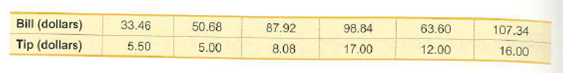# 107.3463.60Bill (dollars)Tip (dollars)50.6898.8433.4687.9216.0012.0017.005.005.508.08

Question
1 views

Tips Using the bill/tip data, find the best predicted tip amount for a dinner bill of \$100. What tipping rule does the regression equation suggest?help_outlineImage Transcriptionclose107.34 63.60 Bill (dollars) Tip (dollars) 50.68 98.84 33.46 87.92 16.00 12.00 17.00 5.00 5.50 8.08 fullscreen
check_circle

Step 1

Regression:

The regression analysis is conducted here by using EXCEL. The software procedure is given below:

• Enter the data.
• Select Data > Data Analysis > Regression> OK.
• Enter Input Y Range as Tip (dollars).
• Enter Input X Range as Bill (dollars).
• Click OK.

The output using EXCEL is as follows:

Step 2

From the output, regression equation is, Tip = –0.35+0.15(Bill).

Strategy for Predicting the y value:

Analyze the below mentioned conditions for predicting the y value.

• In scatterplot, the regression line fits the point well.
• The value of r indicates that there is a linear correlation between the variables.
• The prediction value is not away from the range of the given sample data.

If the regression equation satisfies the above mentioned conditions, then the regression is said to be a good model. Here, substitute the given value of x into the regression equation to predict the value of y.

If the regression equation does not satisfy the above conditions, then the regression is not a good model. Here, the best predicted value of y is the value of.

The scatter diagram, along with the best fitted line (least-squares regression line) for the data is as follows:

Step 3

The horizontal axis represents bill and the vertical axis represents tip.

From the plot, it is observed that the regression line fit the points well.

Correlation coefficient r:

The hypotheses are given below:

Null hypothesis:

H0: r = 0

That is, there is no linear correlation between the bill and tip amounts.

Alternative hypothesis:

Ha: r≠ 0

That is, there is a linear correlation between the bill and tip amounts.

Sample correlation coefficient:

The value of the sample correlation coefficient (r) can be obtained using Excel formula.

Enter the function, =CORREL(A2:A7,B2:B7).

Thus, the value of the sample correlation coefficient, r is 0.828.

Conclusion:

The P-value is 0.042 and the level of...

### Want to see the full answer?

See Solution

#### Want to see this answer and more?

Solutions are written by subject experts who are available 24/7. Questions are typically answered within 1 hour.*

See Solution
*Response times may vary by subject and question.
Tagged in

### Other